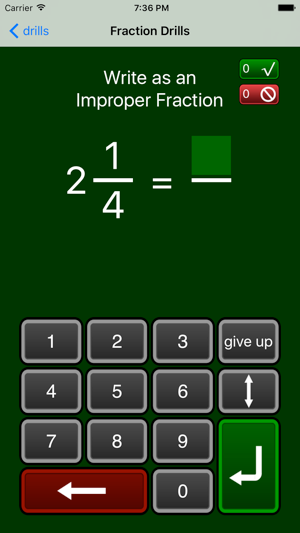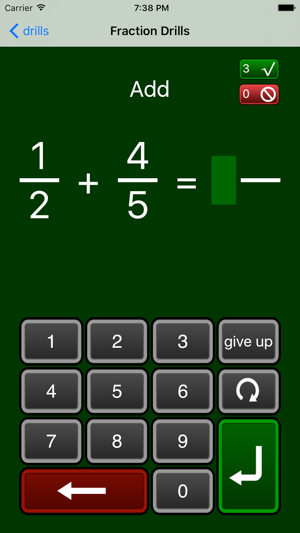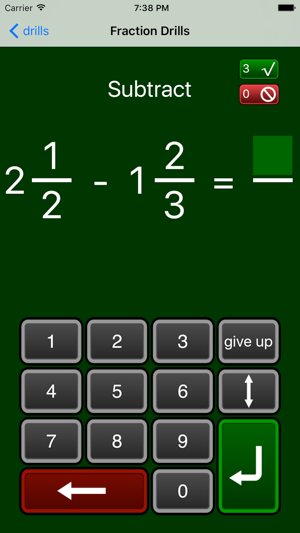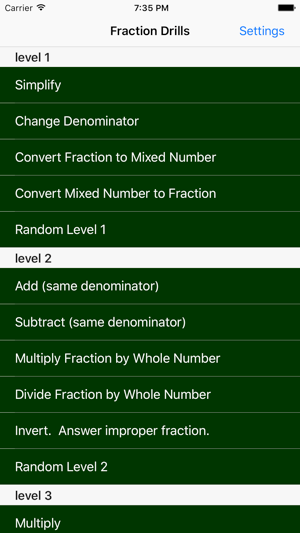## Screenshots

•••••## Description

Having problems learning to work with fractions? Or just want a refresher? This app will help you do that!

The available problem types are organized into "levels", so you can start with easier problems, and work your way up to harder ones. There is a "test" mode that lets you test yourself to see how much you have learned.

Available problem types:

level 1:
* simplify (e.g. 4/6=2/3)
* change denominator (e.g. rewrite 2/3 as 4/6).
* convert fraction to mixed number (e.g. 3/2 is the same as 1 1/2)
* convert mixed number to fraction (rewrite 1 1/2 as 3/2).

Level 2:
* add and subtract (same denominator, e.g. 1/6+2/6)
* multiply and divide by whole numbers (e.g. 2/5*2)
* Invert, and answer with a fraction (e.g. 1 / (2/5) = 5/2 )

Level 3
* Multiply and divide fractions
* Invert, and answer with a mixed number (e.g. 1/ (2/5) = 2 1/2)
* Find the least common denominator (e.g., given 1/4 and 5/6, LCD is 12)

Level 4
* Add mixed numbers (e.g. 1 1/2 + 2 1/2)
* Subtract mixed numbers
* Multiply mixed numbers
* Divide mixed numbers

## What’s New

Version 2.0

Works on lots of screen sizes
Compatible with iOS 10

## Ratings and Reviews

3.7 out of 5
3 Ratings

3 Ratings

Ellayna7 ,

### Doesn't work

Only gives me the same problems over and over

Malia M. ,

### I can't even get this to work and I bought it

I can't even get this to work and I bought it

Ggtkb ,

### Fractions

Wish it harder ones for an eighth grader.

## Information

Seller
William Jockusch
Size
4.2 MB
Category
Education
Compatibility

Requires iOS 8.0 or later. Compatible with iPhone, iPad, and iPod touch.

Languages

English

Age Rating
4+
Price
\$1.99

## Supports

•